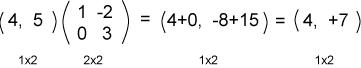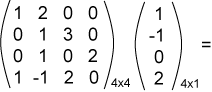# Careful Definitions

You may be uneasy with mathematical operations being defined in this casual fashion. Here are some more accurate definitions:

Matrix times Column Matrix: Let A be a M x C matrix, and x be a C x 1 column matrix. Then the product Ax is the M x 1 column matrix whose i-th element is the dot product of row i of A with x.
Row Matrix times Matrix: Let x be a 1 x R row matrix and A be a R x M matrix. Then the product xA is the 1 x M row matrix whose i-th element is the dot product of x with column i of A.

### QUESTION 12:

Perform the following multiplication: